CLAT  >  Solved Examples on Profit & Loss

# Solved Examples on Profit & Loss - Quantitative Techniques for CLAT

Chapter - 12

PROFIT AND LOSS

The concept of profit and loss is used to study the gain or loss in a business transaction. Whenever we purchase and sell an article we either earn profit or incur loss.

Cost price (CP): The price at which an article is purchased

Sale Price (SP): The price at which an article is sold.

Overhead charges: Apart from the actual cost price, if some charges have to be paid are called overhead charges. Such charges are freight, rent, salary of employees etc.

Profit (If SP > CP): If sale price of a commodity is more than the cost price, some profit is earned.

Loss (If SP < CP): When an article is sold at a price less than the cost price, loss is incurred.

IMPORTANT FORMULAE

1) Profit = Sale price – cost price

P = SP – CP

2) Loss = Cost price – Sale price

L = CP – SP

3) Profit % = (Profit / Cost Price) x 100

= (P / CP) x 100

4) Loss % = (Loss / cost price) x 100

= (L / CP) x 100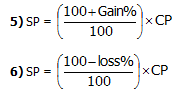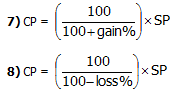Note:

1) Profit or Loss is always on the cost price

2) If an article is sold at a certain gain (say 15%) then SP = 115 % of CP

3) If an article is sold at a certain loss (say 15%)

Then SP = 85% of CP

Example: 1) A person buys an article for Rs. 50 and sells it for Rs. 75. What will be his gain percent?

Solution: CP = Rs. 50     SP = Rs. 75

Gain = 75 – 50 =Rs. 25

We know that gain % = (gain /cp) x 100

= (25 / 50) x 100 = 50%

Example 2: A person buys an umbrella for Rs. 450 and sells it for Rs. 350/-. Find his loss %.

Solution: CP = Rs. 450               SP: Rs. 350

Loss = 450 – 350 =Rs. 100.

Loss percent = (Loss / CP) x 100

= (100 / 450) x 100 = 200/9 % =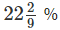Example 3: Find the sale price if CP is Rs. 160 and gain is 20%.

Solutions: SP = 120% of CP

= (120 / 100) x 160 = Rs. 192

Example 4: Find sale price when CP is Rs. 160 and loss is 20%.

Solution: SP = 80% of CP

Or SP = (160 x 80) / 100 = Rs. 128

Example 5: Find CP when SP Rs. 64 and loss is 20%

Solution: CP =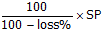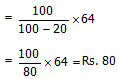Example 6: Find CP when SP = 192 and profit is 20%

Solutions: CP =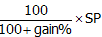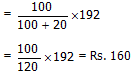Example 7: A chair is bought for Rs. 150 and sold at a gain of 8%. Find the selling price.

Solutions: SP =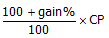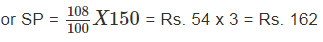Example 8: A bicycle is bought for Rs. 1500 and sold at a loss of 6%. Find the selling price.

Solutions: CP = 1500                 Loss = 6%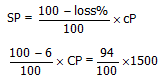Example 9: A table is sold for Rs. 1440 and there is a loss of 10%. At what price should it be sold to gain 10%.

Solutions: SP = Rs. 1440                        Loss = 10%

So CP =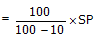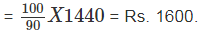To find SP to gain 10%

CP = Rs. 1600    profit = 10%

SP =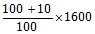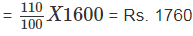Example 10: Ravi lost 20% by selling a watch at Rs. 1536. What will be his gain percent if he sells if for Rs. 2040.

Solution: SP = Rs. 1536             Loss = 20%

CP =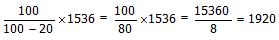Now SP = Rs 2040, CP = 1920, gain = 2040 – 1920 = Rs 120

Gain percent =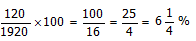Example 11: A shopkeeper sells an article at a profit of 20%. If he had bought it at 20% less and sold for Rs. 10 less, he would have gained 25%. Find the cost price of the article.

Solution: Let CP be 100              SP= 120

If the CP was 100 – 20 = Rs 80 and gain 25%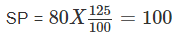Old SP – New SP = Rs. 120 – 100 = Rs. 20

If there is a different of Rs. 20     CP = 100

Different of Rs. 10 CP = 50

Example 12: A person sells apples at 10 for a rupee and gains 20%. How many apples did he buy for a rupee.

Solution: In questions where some quantity of some article is bought or sold, profit or loss is on the quantity sold

Quantity sold is 10 apples

Profit = 20%

Profit on 10 apple is 20%

So profit =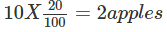It means he has saved 2 apples after selling 10. So he bought 12 for a rupee.

Example 13: A shopkeeper mixes 160 kg of rice at Rs. 27 per kg with 240 kg of rice at Rs. 32 per kg and sells the mixture to gain 20%. What is the sale rate of the mixture.

Solutions: Cost of 160 kg of rice @ Rs. 27 per kg.

= 160 x 27 = Rs. 4320

Cost of Rs. 240 kg of rice @ Rs. 32 per kg = 240 x 32 = 7680

Total = 7680 + 4320 = 12000

Now CP = Rs. 12000

Gain = 20%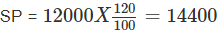So sale price kg =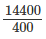Rs. 36 per kg

Example 14: A man buys certain no. of oranges at the rate of 3 per rupee and the same number at 4 per rupee. He mixes then together and sells at 7 for two rupees. Find his gain or loss%.

Solution: LCM of 3 and 4 is = 12

Let us suppose he purchases

12 oranges at the rate of 3 a rupee. Money spent = Rs. 4

and 12 oranges at the rate of 4 a rupee = money spent = Rs. 3

so total oranges purchased = 24

and CP = Rs. 7

now he sells 24 oranges at the rate of 7 for Rs. 2

SP =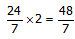Loss = CP – SP = 7 -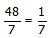% loss =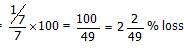Technique to solve problems in a fast way

If a person sells two articles, one at a loss of x % and another at a gain of x%. then there is always a loss to the seller and the loss is equal to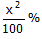Example 15: A man sells two horses for Rs. 4000 each. On one of the horses he loses 20% while on the other he gains 20%. Find his gain / loss percent in the whole transaction.

Solutions: Loss =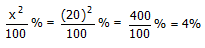Example 16: The profit on selling an article for Rs. 1196 is equal to the loss on selling the same article for Rs. 1056. The cost price of the article is:

1) 1143                                     2) 1134

3) 1156                                     4) 1126

5) None of these

Solutions: Since gain and loss on selling the same article is equal, the cost price must lie at the middle of two sale prices.

On CP =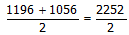= Rs. 1126

Example 17: On selling an article for Rs. 264 a man loses 4%. He should sell the article for how much so that his gain is 12%.

Solution: SP is given to be = Rs. 264

Loss = 4%

So CP =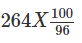Now the gain he wants is 12%

So the SP =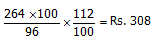Example 18: If the selling price of 10 pens is the same as the cost price of 8 pens. Find the gain or loss percent.

Solution: In such like questions, when cost price and the selling price is the same and quantities sold and purchased is given, the profit / loss is on the quantity sold.

For some money he purchased 8 pens and sold 10 pens for the same amount. It means he lost 2 pens.

So on 10 pens he has lost 2 pens. on 100, he has lost

2/10 x 100 = 20 pens

So los is 20%

Example 19: On selling 100 mangoes, a person gains the SP of 20 mangoes. Find his gain percent.

Solution: Let the SP of 100 mangoes be Rs. 100

He saves the SP of 20 mangoes which is equal to Rs. 20

So gain = Rs. 20

CP = Rs. 100 – Rs. 20 = Rs. 80

Gain % = 20/80 x 100 = 25%

Example 20: On selling a shaving machine for Rs. 1530, the loss is 10%. What will be the gain percent if the machine is sold for Rs. 1819.

Solutions: 1st SP = Rs. 1530

Loss = 10%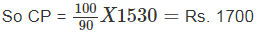Now 2nd SP = Rs. 1819

Gain = Rs. 1819 – Rs. 1700 = s. 119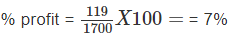Example 21: A trader allows a discount of 15% of the marked price on his article. How much above the cost price should he mark the price to gain 19%.

Solution: let the marked price be Rs. 100.

Discount = 15%

SP = 100 – 15= 85

He wants to make a profit of 19%

So his CP should be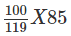If the CP is 8500/119 he should mark Rs. 100

If the CP is 1 he should mark Rs. 100 x (119 / 8500)

If the CP is 100 he should mark Rs. =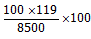He should mark the price 40% more than the CP.

Example 22: I purchased an item for Rs. 8200 and sold it at a gain of 25%. From the sale price of the item I purchased another item and sold it at a loss of 20%. What will be the overall Gain or loss.

Solutions: CP = Rs. 8200

Gain = 25%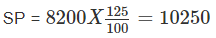Again CP = 10250

Loss % = 20%So there will not be any loss or gain.

Example 23: The cost price of an item is two third of its selling price. What is the gain / loss percent?

Solution:  let CP = Rs. 100

Then SP =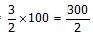Gain =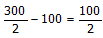Gain % =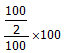= 50%

Example 24: Apples are bought at 6 for Rs. 5 and sold at 8 for Rs. 11. Find the gain or loss per cent.

Solution: Let the no. of apples purchased and sold be 24 (LCM of 6 and 8)

CP of 24 apples = Rs. 20

SP of 24 apples = Rs. 33

Gain = Rs. 33 – Rs. 20 = Rs. 13

So gain % =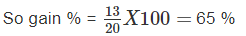Example 25: Find a single discount equivalent to three successive discounts of 10%, 20% and 40%.

Solution: On Rs. 100, 10% discount is given so SP = 100 – 10 = 90

20% discount on Rs. 90 =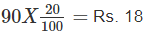The balance amount is 90 – 18 = 72

40% of discount on Rs 72 =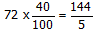Balance amount is 72 -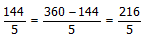Total discount = 100 -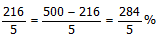= 56.8%

The document Solved Examples on Profit & Loss | Quantitative Techniques for CLAT is a part of the CLAT Course Quantitative Techniques for CLAT.
All you need of CLAT at this link: CLAT

## Quantitative Techniques for CLAT

46 videos|69 docs|95 tests

## FAQs on Solved Examples on Profit & Loss - Quantitative Techniques for CLAT

 1. What is the formula to calculate profit?Ans. The formula to calculate profit is: Profit = Selling Price - Cost Price
 2. How is profit percentage calculated?Ans. Profit percentage is calculated using the following formula: Profit Percentage = (Profit / Cost Price) * 100
 3. What is the difference between profit and loss?Ans. Profit occurs when the selling price of a product is higher than its cost price, resulting in a positive gain. On the other hand, loss occurs when the selling price is lower than the cost price, resulting in a negative gain.
 4. How can I calculate the cost price if the selling price and profit percentage are given?Ans. To calculate the cost price, you can use the following formula: Cost Price = (Selling Price * 100) / (100 + Profit Percentage)
 5. Can you give an example of a profit and loss calculation?Ans. Sure! Let's say the cost price of a product is \$50 and the selling price is \$70. To calculate the profit, we can use the formula: Profit = Selling Price - Cost Price = \$70 - \$50 = \$20 Now, let's calculate the profit percentage using the formula: Profit Percentage = (Profit / Cost Price) * 100 = (\$20 / \$50) * 100 = 40% In this example, the profit is \$20, and the profit percentage is 40%.

## Quantitative Techniques for CLAT

46 videos|69 docs|95 testsExplore Courses for CLAT examSignup to see your scores go up within 7 days! Learn & Practice with 1000+ FREE Notes, Videos & Tests.
10M+ students study on EduRev
Track your progress, build streaks, highlight & save important lessons and more!
Related Searches

,

,

,

,

,

,

,

,

,

,

,

,

,

,

,

,

,

,

,

,

,

;